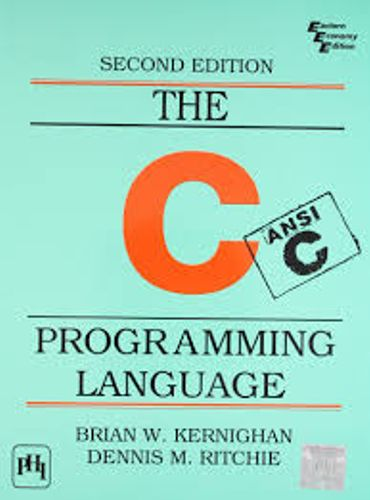# Variables and Types

### Data types

C has several types of variables, but there are a few basic types:

• Integers – whole numbers which can be either positive or negative. Defined using `char, int, short, long or long long`.
• Unsigned integers – whole numbers which can only be positive. Defined using `unsigned char, unsigned int, unsigned short, unsigned long or unsigned long long.`
• Floating point numbers – real numbers (numbers with fractions). Defined using `float `and` double.`
• Structures – will be explained later, in the Structures section.

The different types of variables define their bounds. A `char` can range only from -128 to 127, whereas a `long` can range from -2,147,483,648 to 2,147,483,647 (`long` and other numeric data types may have another range on different computers, for example – from –9,223,372,036,854,775,808 to 9,223,372,036,854,775,807 on 64-bit computer).

Note that C does not have a boolean type. Usually, it is defined using the following notation:

```#define BOOL char
#define FALSE 0
#define TRUE 1
```

C uses arrays of characters to define strings, and will be explained in the Strings section.

### Defining variables

For numbers, we will usually use the type `int`, which an integer in the size of a “word” the default number size of the machine which your program is compiled on. On most computers today, it is a 32-bit number, which means the number can range from -2,147,483,648 to 2,147,483,647.

To define the variables `foo` and `bar`, we need to use the following syntax:

```int foo;
int bar = 1;
```

The variable `foo` can be used, but since we did not initialize it, we don’t know what’s in it. The variable `bar` contains the number 1.

Now, we can do some math. Assuming `a``b``c``d`, and `e` are variables, we can simply use plus, minus and multiplication operators in the following notation, and assign a new value to `a`:

```int a = 0, b = 1, c = 2, d = 3, e = 4;
a = b - c + d * e;
printf("%d", a); /* will print 1-2+3*4 = 11 */
```

## Home Work

In the next tutorial, you will need to create a program which prints out the sum of the numbers x, `y`, and `z`. Start Exercise

Click on image to Download C Programming Language Book :